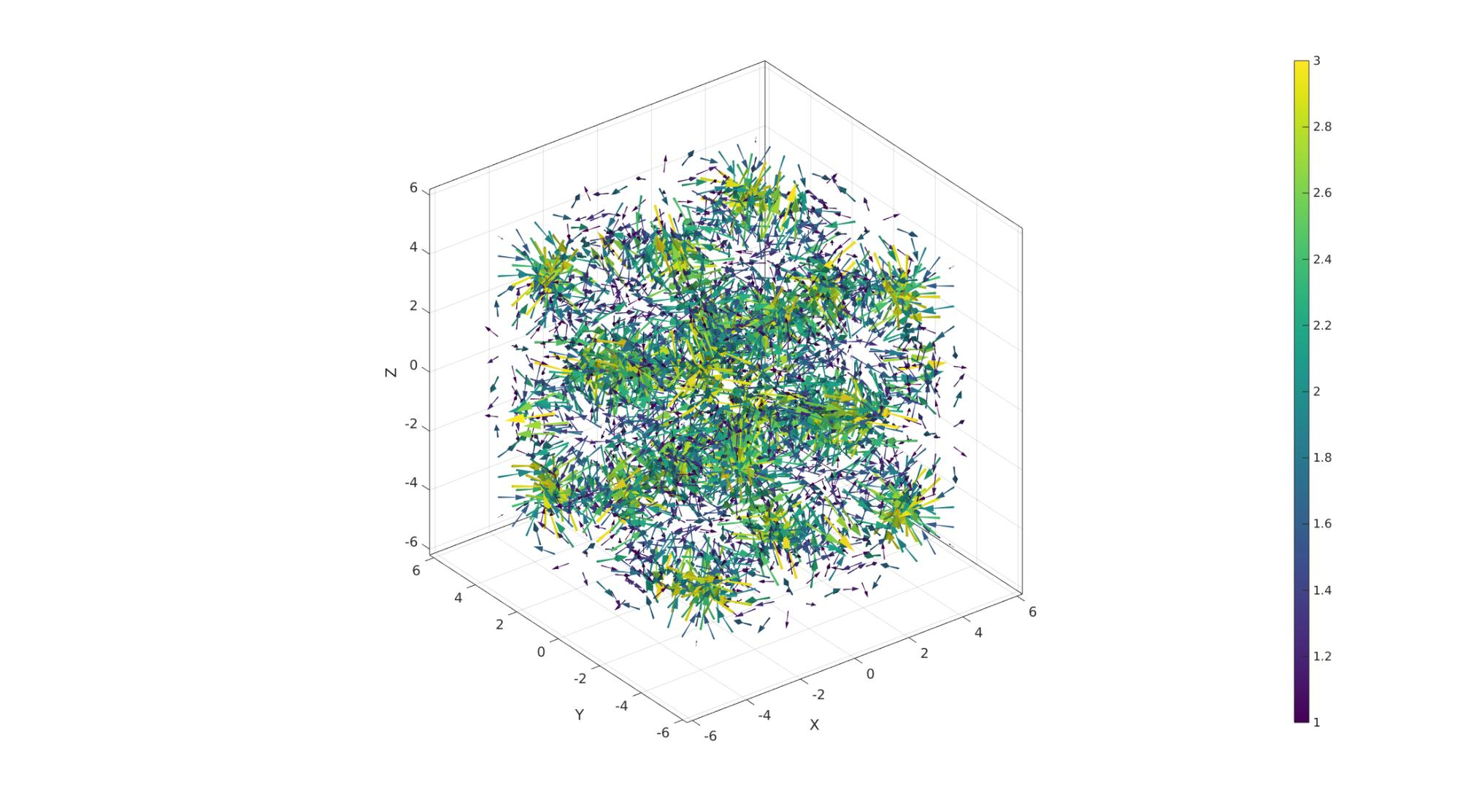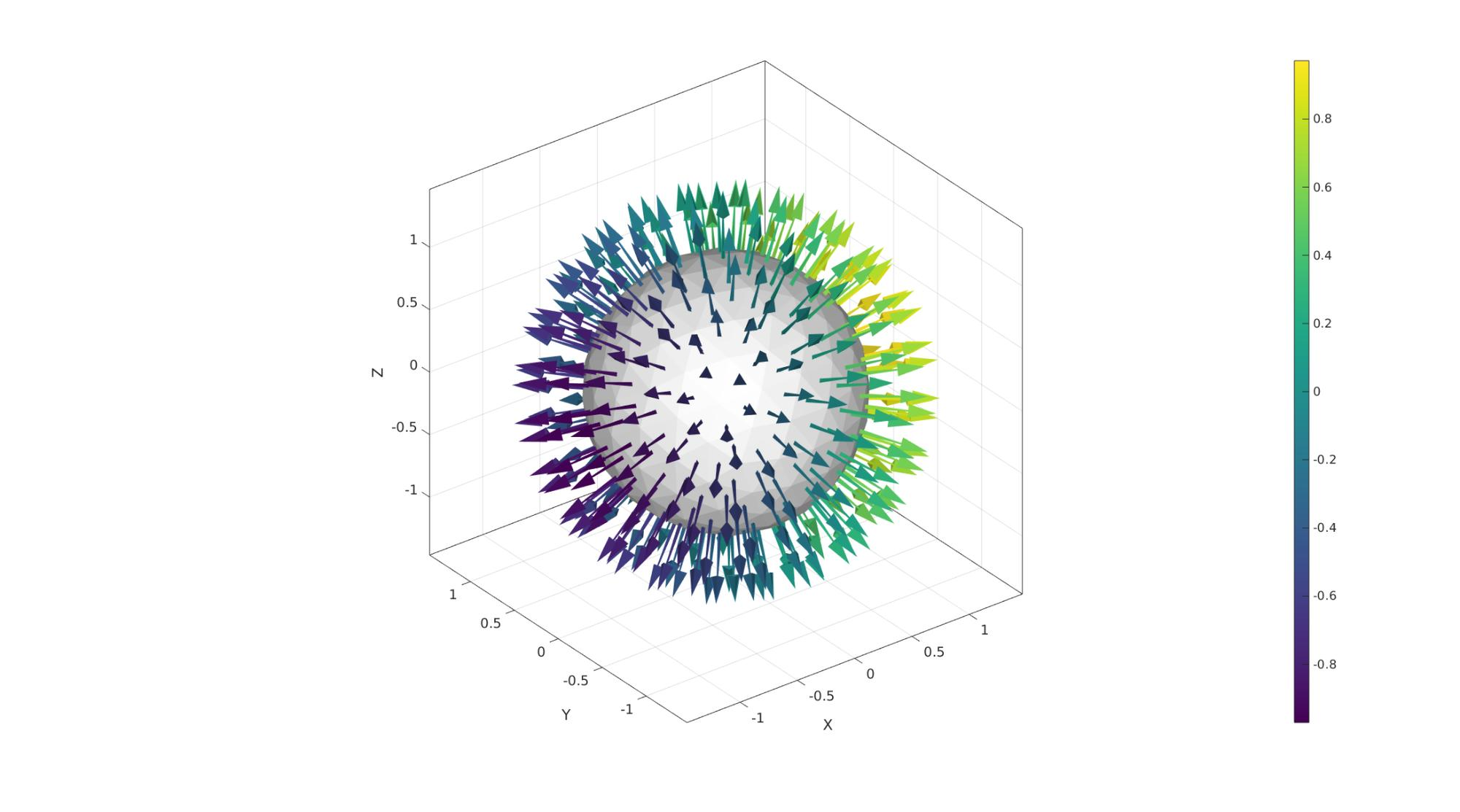# quiverVec

Below is a demonstration of the features of the quiverVec function

## Contents

```clear; close all; clc;
```

## Syntax

|[hp]=quiverVec(P,V,vecSize,colorSpec,edgeColorOpt,quiverStyleOpt,alphaLevel);

## Description

This function is a short-hand version of the quiver3Dpatch function which enables colormapped and 3D rendering of directional or vector data. The inputs are: P: The vector origins V: The vectors vecSize: The vector sizes colorSpec: The color data which is a string, rgb values or colormapped arrays edgeColorOpt: Edge coloring of the vector quiverStyleOpt: The quiver style, which is 1=normal arrow, 2=Arrive at origin, 3=Pass through origin, 4=Two-sided. alphaLevel: the transparancy level

## Examples

```clear; close all; clc;
```

Plot settings

```cMap=viridis(250);
fontSize=15;
```

## Example for multidimensional image data: colormap driven vectors

Simulating 3D volume and vector data

```n=15;
[X,Y,Z]=meshgrid(linspace(-4.77,4.77,n));
phi=(1+sqrt(5))/2;
M=2 - (cos(X + phi*Y) + cos(X - phi*Y) + cos(Y + phi*Z) + cos(Y - phi*Z) + cos(Z - phi*X) + cos(Z + phi*X));
C=hypot(hypot(u(:),v(:)),w(:)); %Vector lenghts
P=[X(:) Y(:) Z(:)];
V=[u(:) v(:) w(:)];
```
```cFigure;

quiverVec(P,V,C,C);

colormap(cMap); colorbar;
axisGeom(gca,fontSize);
clim([1 3])
drawnow;
```## Example for face normal plotting with variable color

Creating a surface model

```[F,V]=geoSphere(2,1);
[N,Vn]=patchNormal(F,V); %Get face normals
Cn=Vn(:,1); %Color data
```
```cFigure;

gpatch(F,V,'w','none');
quiverVec(Vn,N,0.5,Cn);

colormap(cMap); colorbar;
axisGeom(gca,fontSize);
drawnow;
```GIBBON www.gibboncode.org

Kevin Mattheus Moerman, [email protected]

GIBBON footer text

GIBBON: The Geometry and Image-based Bioengineering add-On. A toolbox for image segmentation, image-based modeling, meshing, and finite element analysis.

Copyright (C) 2006-2023 Kevin Mattheus Moerman and the GIBBON contributors

This program is free software: you can redistribute it and/or modify it under the terms of the GNU General Public License as published by the Free Software Foundation, either version 3 of the License, or (at your option) any later version.

This program is distributed in the hope that it will be useful, but WITHOUT ANY WARRANTY; without even the implied warranty of MERCHANTABILITY or FITNESS FOR A PARTICULAR PURPOSE. See the GNU General Public License for more details.

You should have received a copy of the GNU General Public License along with this program. If not, see http://www.gnu.org/licenses/.